## 5.157. full_group

Origin
Constraint

$\mathrm{𝚏𝚞𝚕𝚕}_\mathrm{𝚐𝚛𝚘𝚞𝚙}\left(\begin{array}{c}\mathrm{𝙽𝙶𝚁𝙾𝚄𝙿},\hfill \\ \mathrm{𝙼𝙸𝙽}_\mathrm{𝚂𝙸𝚉𝙴},\hfill \\ \mathrm{𝙼𝙰𝚇}_\mathrm{𝚂𝙸𝚉𝙴},\hfill \\ \mathrm{𝙼𝙸𝙽}_\mathrm{𝙳𝙸𝚂𝚃},\hfill \\ \mathrm{𝙼𝙰𝚇}_\mathrm{𝙳𝙸𝚂𝚃},\hfill \\ \mathrm{𝙽𝚅𝙰𝙻},\hfill \\ \mathrm{𝚅𝙰𝚁𝙸𝙰𝙱𝙻𝙴𝚂},\hfill \\ \mathrm{𝚅𝙰𝙻𝚄𝙴𝚂}\hfill \end{array}\right)$

Synonym

$\mathrm{𝚐𝚛𝚘𝚞𝚙}_\mathrm{𝚠𝚒𝚝𝚑𝚘𝚞𝚝}_\mathrm{𝚋𝚘𝚛𝚍𝚎𝚛}$.

Arguments
 $\mathrm{𝙽𝙶𝚁𝙾𝚄𝙿}$ $\mathrm{𝚍𝚟𝚊𝚛}$ $\mathrm{𝙼𝙸𝙽}_\mathrm{𝚂𝙸𝚉𝙴}$ $\mathrm{𝚍𝚟𝚊𝚛}$ $\mathrm{𝙼𝙰𝚇}_\mathrm{𝚂𝙸𝚉𝙴}$ $\mathrm{𝚍𝚟𝚊𝚛}$ $\mathrm{𝙼𝙸𝙽}_\mathrm{𝙳𝙸𝚂𝚃}$ $\mathrm{𝚍𝚟𝚊𝚛}$ $\mathrm{𝙼𝙰𝚇}_\mathrm{𝙳𝙸𝚂𝚃}$ $\mathrm{𝚍𝚟𝚊𝚛}$ $\mathrm{𝙽𝚅𝙰𝙻}$ $\mathrm{𝚍𝚟𝚊𝚛}$ $\mathrm{𝚅𝙰𝚁𝙸𝙰𝙱𝙻𝙴𝚂}$ $\mathrm{𝚌𝚘𝚕𝚕𝚎𝚌𝚝𝚒𝚘𝚗}\left(\mathrm{𝚟𝚊𝚛}-\mathrm{𝚍𝚟𝚊𝚛}\right)$ $\mathrm{𝚅𝙰𝙻𝚄𝙴𝚂}$ $\mathrm{𝚌𝚘𝚕𝚕𝚎𝚌𝚝𝚒𝚘𝚗}\left(\mathrm{𝚟𝚊𝚕}-\mathrm{𝚒𝚗𝚝}\right)$
Restrictions
 $\mathrm{𝙽𝙶𝚁𝙾𝚄𝙿}\ge 0$ $\mathrm{𝙼𝙸𝙽}_\mathrm{𝚂𝙸𝚉𝙴}\ge 0$ $\mathrm{𝙼𝙰𝚇}_\mathrm{𝚂𝙸𝚉𝙴}\ge \mathrm{𝙼𝙸𝙽}_\mathrm{𝚂𝙸𝚉𝙴}$ $\mathrm{𝙼𝙸𝙽}_\mathrm{𝙳𝙸𝚂𝚃}\ge 0$ $\mathrm{𝙼𝙰𝚇}_\mathrm{𝙳𝙸𝚂𝚃}\ge \mathrm{𝙼𝙸𝙽}_\mathrm{𝙳𝙸𝚂𝚃}$ $\mathrm{𝙼𝙰𝚇}_\mathrm{𝙳𝙸𝚂𝚃}\le |\mathrm{𝚅𝙰𝚁𝙸𝙰𝙱𝙻𝙴𝚂}|-2$ $\mathrm{𝙽𝚅𝙰𝙻}\ge \mathrm{𝙼𝙰𝚇}_\mathrm{𝚂𝙸𝚉𝙴}$ $\mathrm{𝙽𝚅𝙰𝙻}\ge \mathrm{𝙽𝙶𝚁𝙾𝚄𝙿}$ $\mathrm{𝙽𝚅𝙰𝙻}\le |\mathrm{𝚅𝙰𝚁𝙸𝙰𝙱𝙻𝙴𝚂}|-2$ $\mathrm{𝚛𝚎𝚚𝚞𝚒𝚛𝚎𝚍}$$\left(\mathrm{𝚅𝙰𝚁𝙸𝙰𝙱𝙻𝙴𝚂},\mathrm{𝚟𝚊𝚛}\right)$ $\mathrm{𝚛𝚎𝚚𝚞𝚒𝚛𝚎𝚍}$$\left(\mathrm{𝚅𝙰𝙻𝚄𝙴𝚂},\mathrm{𝚟𝚊𝚕}\right)$ $\mathrm{𝚍𝚒𝚜𝚝𝚒𝚗𝚌𝚝}$$\left(\mathrm{𝚅𝙰𝙻𝚄𝙴𝚂},\mathrm{𝚟𝚊𝚕}\right)$
Purpose

Let $n$ be the number of variables of the collection $\mathrm{𝚅𝙰𝚁𝙸𝙰𝙱𝙻𝙴𝚂}$. Let ${X}_{i},{X}_{i+1},\cdots ,{X}_{j}$ $\left(1\le i\le j\le n\right)$ be consecutive variables of the collection of variables $\mathrm{𝚅𝙰𝚁𝙸𝙰𝙱𝙻𝙴𝚂}$ such that all the following conditions simultaneously apply:

• All variables ${X}_{i},\cdots ,{X}_{j}$ take their value in the set of values $\mathrm{𝚅𝙰𝙻𝚄𝙴𝚂}$,

• $i=1$ or ${X}_{i-1}$ does not take a value in $\mathrm{𝚅𝙰𝙻𝚄𝙴𝚂}$,

• $j=n$ or ${X}_{j+1}$ does not take a value in $\mathrm{𝚅𝙰𝙻𝚄𝙴𝚂}$.

We call such a sequence of variables a group. A full group is a group that neither starts at position 1 nor ends at position $n$. Similarly an anti-full group is a maximum sequence of variables that are not assigned any value from $\mathrm{𝚅𝙰𝙻𝚄𝙴𝚂}$ that neither starts at position 1 nor ends at position $n$.

The constraint $\mathrm{𝚏𝚞𝚕𝚕}_\mathrm{𝚐𝚛𝚘𝚞𝚙}$ is true if all the following conditions hold:

• There are exactly $\mathrm{𝙽𝙶𝚁𝙾𝚄𝙿}$ full groups of variables,

• $\mathrm{𝙼𝙸𝙽}_\mathrm{𝚂𝙸𝚉𝙴}$ is the number of variables of the smallest full group,

• $\mathrm{𝙼𝙰𝚇}_\mathrm{𝚂𝙸𝚉𝙴}$ is the number of variables of the largest full group,

• $\mathrm{𝙼𝙸𝙽}_\mathrm{𝙳𝙸𝚂𝚃}$ is the number of variables of the smallest anti-full group,

• $\mathrm{𝙼𝙰𝚇}_\mathrm{𝙳𝙸𝚂𝚃}$ is the number of variables of the largest anti-full group,

• $\mathrm{𝙽𝚅𝙰𝙻}$ is the number of variables that belong to a full group.

Example
$\left(2,2,3,1,1,5,〈0,1,2,6,2,7,4,8,9〉,〈0,2,4,6,8〉\right)$

Given the fact that full groups are formed by even values in $\left\{0,2,4,6,8\right\}$ (i.e., values expressed by the $\mathrm{𝚅𝙰𝙻𝚄𝙴𝚂}$ collection), the $\mathrm{𝚏𝚞𝚕𝚕}_\mathrm{𝚐𝚛𝚘𝚞𝚙}$ constraint holds since:

• Its first argument, $\mathrm{𝙽𝙶𝚁𝙾𝚄𝙿}$, is set to value 2 since the sequence $012627489$ contains two full groups of even values (i.e., group $262$ and group $48$). Note that the first 0 is not a full group since it is located at the first position of the sequence.

• Its second argument, $\mathrm{𝙼𝙸𝙽}_\mathrm{𝚂𝙸𝚉𝙴}$, is set to value 2 since the smallest full group of even values involves only two elements (i.e., the full group $48$).

• Its third argument, $\mathrm{𝙼𝙰𝚇}_\mathrm{𝚂𝙸𝚉𝙴}$, is set to value 3 since the largest full group of even values involves three elements (i.e., the full group $262$).

• Its fourth argument, $\mathrm{𝙼𝙸𝙽}_\mathrm{𝙳𝙸𝚂𝚃}$, is set to value 1 since the smallest anti-full groups involve a single element (i.e., the anti-full groups 1 and 7).

• Its fifth argument, $\mathrm{𝙼𝙰𝚇}_\mathrm{𝙳𝙸𝚂𝚃}$, is set to value 1 since the largest anti-full groups involve a single element (i.e., the anti-full groups 1 and 7).

• Its sixth argument, $\mathrm{𝙽𝚅𝙰𝙻}$, is set to value 5 since the total number of even values part of a full group of the sequence $012627489$ is equal to 5 (i.e., elements 2, 6, 2, 4 and 8).

Typical
 $\mathrm{𝙽𝙶𝚁𝙾𝚄𝙿}>0$ $\mathrm{𝙼𝙸𝙽}_\mathrm{𝚂𝙸𝚉𝙴}>0$ $\mathrm{𝙼𝙰𝚇}_\mathrm{𝚂𝙸𝚉𝙴}>\mathrm{𝙼𝙸𝙽}_\mathrm{𝚂𝙸𝚉𝙴}$ $\mathrm{𝙼𝙸𝙽}_\mathrm{𝙳𝙸𝚂𝚃}>0$ $\mathrm{𝙼𝙰𝚇}_\mathrm{𝙳𝙸𝚂𝚃}>\mathrm{𝙼𝙸𝙽}_\mathrm{𝙳𝙸𝚂𝚃}$ $\mathrm{𝙼𝙰𝚇}_\mathrm{𝙳𝙸𝚂𝚃}<|\mathrm{𝚅𝙰𝚁𝙸𝙰𝙱𝙻𝙴𝚂}|$ $\mathrm{𝙽𝚅𝙰𝙻}>\mathrm{𝙼𝙰𝚇}_\mathrm{𝚂𝙸𝚉𝙴}$ $\mathrm{𝙽𝚅𝙰𝙻}>\mathrm{𝙽𝙶𝚁𝙾𝚄𝙿}$ $\mathrm{𝙽𝚅𝙰𝙻}<|\mathrm{𝚅𝙰𝚁𝙸𝙰𝙱𝙻𝙴𝚂}|$ $|\mathrm{𝚅𝙰𝚁𝙸𝙰𝙱𝙻𝙴𝚂}|>1$ $\mathrm{𝚛𝚊𝚗𝚐𝚎}$$\left(\mathrm{𝚅𝙰𝚁𝙸𝙰𝙱𝙻𝙴𝚂}.\mathrm{𝚟𝚊𝚛}\right)>1$ $|\mathrm{𝚅𝙰𝙻𝚄𝙴𝚂}|>0$ $|\mathrm{𝚅𝙰𝚁𝙸𝙰𝙱𝙻𝙴𝚂}|>|\mathrm{𝚅𝙰𝙻𝚄𝙴𝚂}|$
Symmetries
• Items of $\mathrm{𝚅𝙰𝚁𝙸𝙰𝙱𝙻𝙴𝚂}$ can be reversed.

• Items of $\mathrm{𝚅𝙰𝙻𝚄𝙴𝚂}$ are permutable.

• An occurrence of a value of $\mathrm{𝚅𝙰𝚁𝙸𝙰𝙱𝙻𝙴𝚂}.\mathrm{𝚟𝚊𝚛}$ that belongs to $\mathrm{𝚅𝙰𝙻𝚄𝙴𝚂}.\mathrm{𝚟𝚊𝚕}$ (resp. does not belong to $\mathrm{𝚅𝙰𝙻𝚄𝙴𝚂}.\mathrm{𝚟𝚊𝚕}$) can be replaced by any other value in $\mathrm{𝚅𝙰𝙻𝚄𝙴𝚂}.\mathrm{𝚟𝚊𝚕}$ (resp. not in $\mathrm{𝚅𝙰𝙻𝚄𝙴𝚂}.\mathrm{𝚟𝚊𝚕}$).

Arg. properties
• Functional dependency: $\mathrm{𝙽𝙶𝚁𝙾𝚄𝙿}$ determined by $\mathrm{𝚅𝙰𝚁𝙸𝙰𝙱𝙻𝙴𝚂}$ and $\mathrm{𝚅𝙰𝙻𝚄𝙴𝚂}$.

• Functional dependency: $\mathrm{𝙼𝙸𝙽}_\mathrm{𝚂𝙸𝚉𝙴}$ determined by $\mathrm{𝚅𝙰𝚁𝙸𝙰𝙱𝙻𝙴𝚂}$ and $\mathrm{𝚅𝙰𝙻𝚄𝙴𝚂}$.

• Functional dependency: $\mathrm{𝙼𝙰𝚇}_\mathrm{𝚂𝙸𝚉𝙴}$ determined by $\mathrm{𝚅𝙰𝚁𝙸𝙰𝙱𝙻𝙴𝚂}$ and $\mathrm{𝚅𝙰𝙻𝚄𝙴𝚂}$.

• Functional dependency: $\mathrm{𝙼𝙸𝙽}_\mathrm{𝙳𝙸𝚂𝚃}$ determined by $\mathrm{𝚅𝙰𝚁𝙸𝙰𝙱𝙻𝙴𝚂}$ and $\mathrm{𝚅𝙰𝙻𝚄𝙴𝚂}$.

• Functional dependency: $\mathrm{𝙼𝙰𝚇}_\mathrm{𝙳𝙸𝚂𝚃}$ determined by $\mathrm{𝚅𝙰𝚁𝙸𝙰𝙱𝙻𝙴𝚂}$ and $\mathrm{𝚅𝙰𝙻𝚄𝙴𝚂}$.

• Functional dependency: $\mathrm{𝙽𝚅𝙰𝙻}$ determined by $\mathrm{𝚅𝙰𝚁𝙸𝙰𝙱𝙻𝙴𝚂}$ and $\mathrm{𝚅𝙰𝙻𝚄𝙴𝚂}$.

Keywords
Automaton

Figures 5.157.15.157.35.157.55.157.75.157.9 and 5.157.11 depict the different automata associated with the $\mathrm{𝚏𝚞𝚕𝚕}_\mathrm{𝚐𝚛𝚘𝚞𝚙}$ constraint. For the automata that respectively compute $\mathrm{𝙽𝙶𝚁𝙾𝚄𝙿}$, $\mathrm{𝙼𝙸𝙽}_\mathrm{𝚂𝙸𝚉𝙴}$, $\mathrm{𝙼𝙰𝚇}_\mathrm{𝚂𝙸𝚉𝙴}$, $\mathrm{𝙼𝙸𝙽}_\mathrm{𝙳𝙸𝚂𝚃}$, $\mathrm{𝙼𝙰𝚇}_\mathrm{𝙳𝙸𝚂𝚃}$ and $\mathrm{𝙽𝚅𝙰𝙻}$ we have a 0-1 signature variable ${𝚂}_{i}$ for each variable ${\mathrm{𝚅𝙰𝚁}}_{i}$ of the collection $\mathrm{𝚅𝙰𝚁𝙸𝙰𝙱𝙻𝙴𝚂}$. The following signature constraint links ${\mathrm{𝚅𝙰𝚁}}_{i}$ and ${𝚂}_{i}$: ${\mathrm{𝚅𝙰𝚁}}_{i}\in \mathrm{𝚅𝙰𝙻𝚄𝙴𝚂}⇔{𝚂}_{i}$.

##### Figure 5.157.1. Automaton for the $\mathrm{𝙽𝙶𝚁𝙾𝚄𝙿}$ argument of the $\mathrm{𝚏𝚞𝚕𝚕}_\mathrm{𝚐𝚛𝚘𝚞𝚙}$ constraint and its glue matrix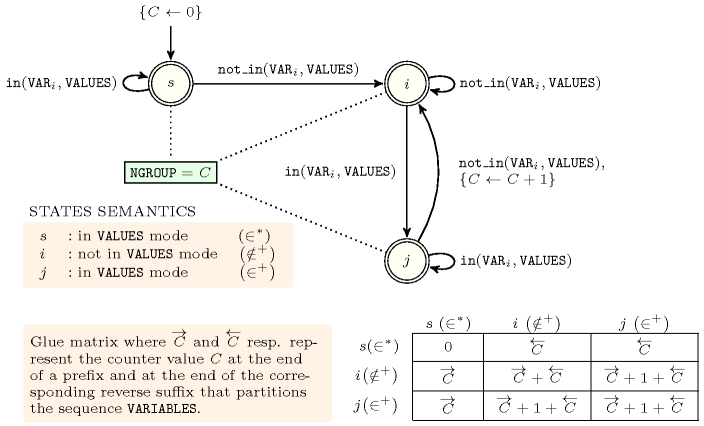##### Figure 5.157.2. Hypergraph of the reformulation corresponding to the automaton (with one counter) of the $\mathrm{𝙽𝙶𝚁𝙾𝚄𝙿}$ argument of the $\mathrm{𝚏𝚞𝚕𝚕}_\mathrm{𝚐𝚛𝚘𝚞𝚙}$ constraint (since all states of the automaton are accepting there is no restriction on the last variable ${Q}_{n}$)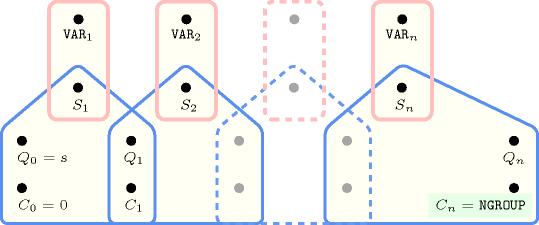##### Figure 5.157.3. Automaton for the $\mathrm{𝙼𝙸𝙽}_\mathrm{𝚂𝙸𝚉𝙴}$ argument of the $\mathrm{𝚏𝚞𝚕𝚕}_\mathrm{𝚐𝚛𝚘𝚞𝚙}$ constraint##### Figure 5.157.4. Hypergraph of the reformulation corresponding to the automaton (with two counters) of the $\mathrm{𝙼𝙸𝙽}_\mathrm{𝚂𝙸𝚉𝙴}$ argument of the $\mathrm{𝚏𝚞𝚕𝚕}_\mathrm{𝚐𝚛𝚘𝚞𝚙}$ constraint (since all states of the automaton are accepting there is no restriction on the last variable ${Q}_{n}$)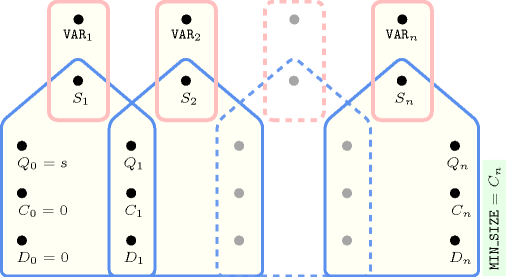##### Figure 5.157.5. Automaton for the $\mathrm{𝙼𝙰𝚇}_\mathrm{𝚂𝙸𝚉𝙴}$ argument of the $\mathrm{𝚏𝚞𝚕𝚕}_\mathrm{𝚐𝚛𝚘𝚞𝚙}$ constraint and its glue matrix##### Figure 5.157.6. Hypergraph of the reformulation corresponding to the automaton (with two counters) of the $\mathrm{𝙼𝙰𝚇}_\mathrm{𝚂𝙸𝚉𝙴}$ argument of the $\mathrm{𝚏𝚞𝚕𝚕}_\mathrm{𝚐𝚛𝚘𝚞𝚙}$ constraint (since all states of the automaton are accepting there is no restriction on the last variable ${Q}_{n}$)##### Figure 5.157.7. Automaton for the $\mathrm{𝙼𝙸𝙽}_\mathrm{𝙳𝙸𝚂𝚃}$ argument of the $\mathrm{𝚏𝚞𝚕𝚕}_\mathrm{𝚐𝚛𝚘𝚞𝚙}$ constraint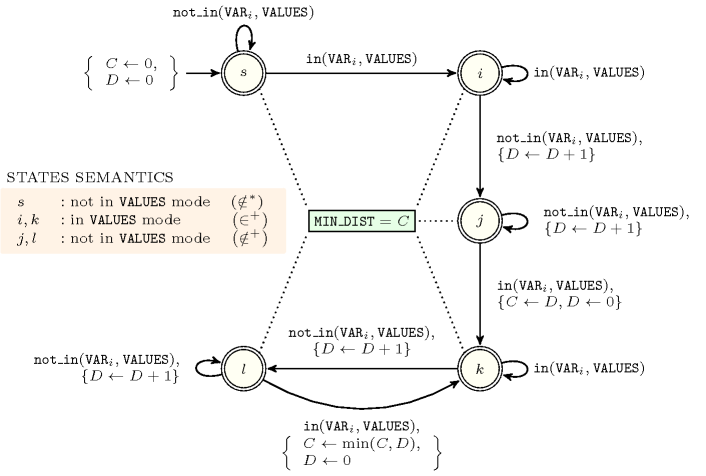##### Figure 5.157.8. Hypergraph of the reformulation corresponding to the automaton (with two counters) of the $\mathrm{𝙼𝙸𝙽}_\mathrm{𝙳𝙸𝚂𝚃}$ argument of the $\mathrm{𝚏𝚞𝚕𝚕}_\mathrm{𝚐𝚛𝚘𝚞𝚙}$ constraint (since all states of the automaton are accepting there is no restriction on the last variable ${Q}_{n}$)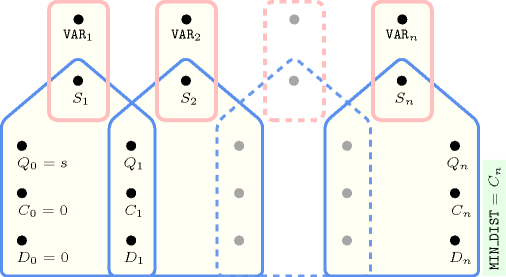##### Figure 5.157.9. Automaton for the $\mathrm{𝙼𝙰𝚇}_\mathrm{𝙳𝙸𝚂𝚃}$ argument of the $\mathrm{𝚏𝚞𝚕𝚕}_\mathrm{𝚐𝚛𝚘𝚞𝚙}$ constraint and its glue matrix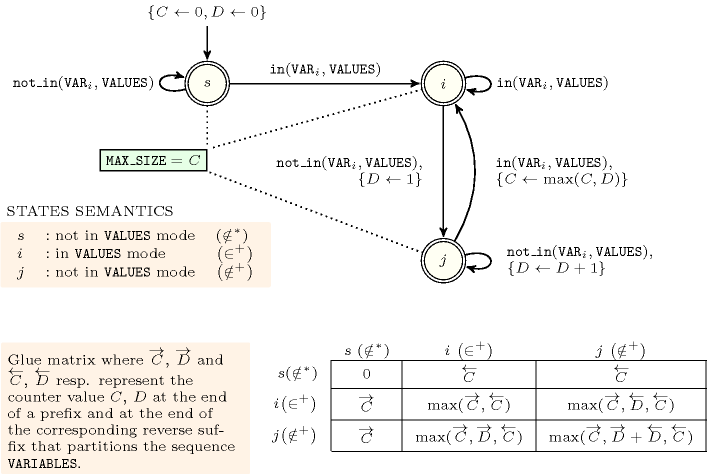##### Figure 5.157.10. Hypergraph of the reformulation corresponding to the automaton (with two counters) of the $\mathrm{𝙼𝙰𝚇}_\mathrm{𝙳𝙸𝚂𝚃}$ argument of the $\mathrm{𝚏𝚞𝚕𝚕}_\mathrm{𝚐𝚛𝚘𝚞𝚙}$ constraint (since all states of the automaton are accepting there is no restriction on the last variable ${Q}_{n}$)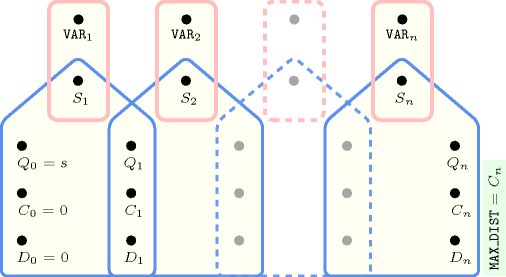##### Figure 5.157.11. Automaton for the $\mathrm{𝙽𝚅𝙰𝙻}$ argument of the $\mathrm{𝚏𝚞𝚕𝚕}_\mathrm{𝚐𝚛𝚘𝚞𝚙}$ constraint and its glue matrix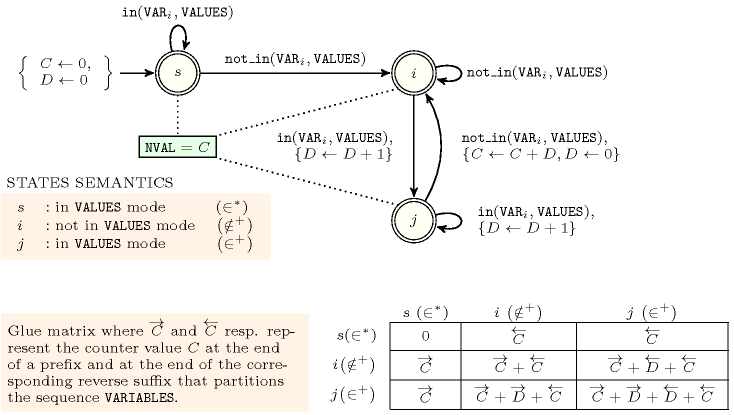##### Figure 5.157.12. Hypergraph of the reformulation corresponding to the automaton (with two counters) of the $\mathrm{𝙽𝚅𝙰𝙻}$ argument of the $\mathrm{𝚏𝚞𝚕𝚕}_\mathrm{𝚐𝚛𝚘𝚞𝚙}$ constraint (since all states of the automaton are accepting there is no restriction on the last variable ${Q}_{n}$)